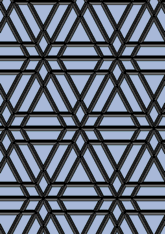data192/TRA0503

## Geometry

• The symmetry group of the tiling is *632 (p6m).
• All the internal angles of the constituent polygons are a multiple of 60°.
• Contains one equilateral triangle.
• Contains one regular two-pointed star polygon with vertex angle of 60°.
• There is one non-regular reflective tile.
• The tiling satisfies the two-colour condition.
• The tiling is edge-to-edge.
• As drawn, contains about 201 polygons.

## References

Publications referenced: